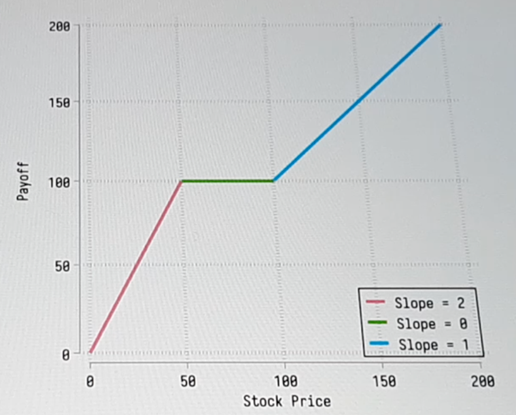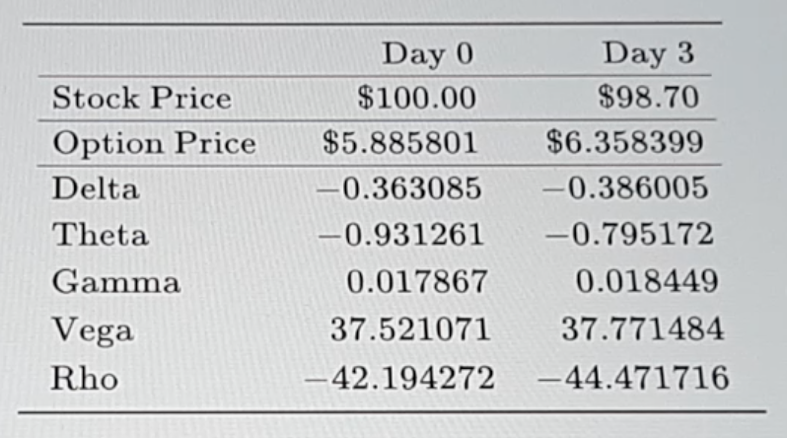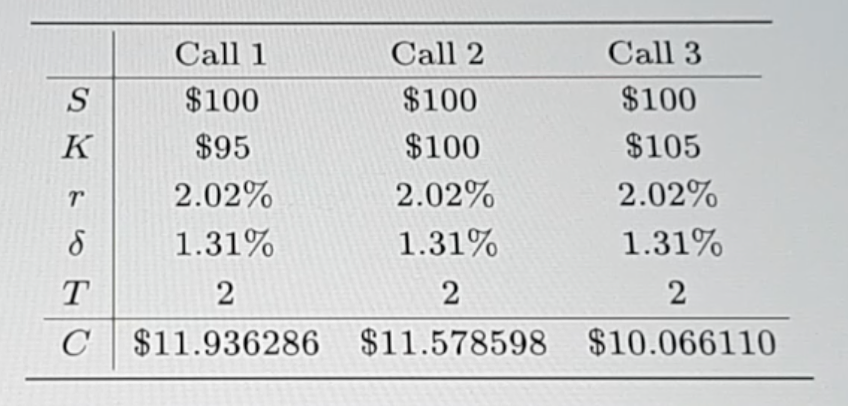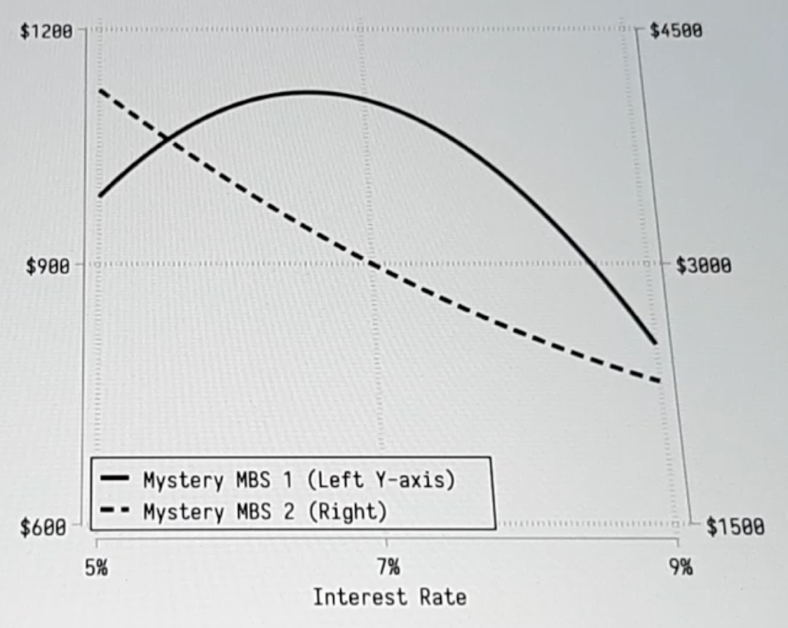Dec 4 / Exam Helper

# Derivative Market- Final Exam Practice Paper

Problem 1
Your firm is offering a new type of exotic equity option. The expiration date of the option is two periods from now. The option is not initially specified to be a put or a call. Instead, the owner makes this choice after one period. Once the choice is made, the option can be exercised at any time. For example, if after one period the owner chooses for the new exotic option to be a put, it would at that time become identical to an ordinary American put with one period remaining until expiration. A customer has asked for a quote for an option of this type on GameGo Inc. stock with a strike price of . The current price of GameGo Inc. stock is  per share, and over each period the stock price evolves as shown on the tree below. The risk-neutral probability of an up move is . The stock does not pay dividends, and the interest rate is  per period (simple compounding).

Let  and  be the values of American calls and puts when the stock price is  and there are  periods to go. (A) What is the value of  and  ?
(B) What is the value of  and  ?
(C) What is the lowest price your firm could charge and still break even?

Problem 2

See the payoff diagram below.Use the following assets to replicate the payoff diagram.

(i) shares of stock
(ii) \$50-strike calls
(iii) \$100-strike puts
(iv) \$100-strike calls

In this question, denote purchased quantities with a positive number and written quantities with a negative number. You may not use bonds.

Problem 3

Below is information (per-share) for a \$102.00-strike purchased put with one year to expiration, where interest rate r=7.13%, volatility σ=0.21, and dividend yield δ=0.00%A dealer sells this put option, on 100 shares. You are to describe the dealer's hedge and evaluate profit or loss after 3 days.

(A) On day 0, how many shares does the dealer buy or sell to delta-hedge the written put? (Fractional shares are permissible.) In this question, denote quantities to buy with a positive number and quantities to sell with a negative number.

(B) On day O, taking into account the option premium received and the share transaction from part (A), what position in the risk-free asset will give the dealer zero net investment? In this question, denote amount to lend with a positive number and amount to borrow with a negative number.

(C) On day 3, what is the dealer's profit on this hedged position? (Use a negative number to denote losses.)

Problem 4
You have the information listed below on three options on a normalized stock price index, including the current index value S, the risk-free rate r, the dividend yield δ, the time to expiration in years T, and the call price C.(A) Based on this information, and using an Excel spreadsheet with the Black-Scholes-Merton model, estimate the implied volatility from each option. Write your answers in decimal numbers.
σ1
σ2
σ3

(B) If you want to take a long-short position that will profit if the implied volatilities of these options tend to converge over time, which of the following positions might you choose? There may be more than one correct answer; indicate all that apply.

• long call 1 and short call 2
• long call 1 and short call 3
• short call 1 and long call 2
• short call 1 and long call 3
• long call 3 and short call 2

Problem 5

The two-year risk-free interest rates in Germany and in the United States are, respectively, 0.11% and 0.93% per annum with continuous compounding. The spot price of the Euro is \$1.15. What is the two-year forward price of \$1.00 in Euros?

Problem 6

Merit Inc. has zero coupon debt outstanding that will have to be repaid in three years, with a face value of \$80.00 million. The risk-free interest rate is 5.13% per annum (continuously compounded). You estimate that the firm's asset volatility is 16.29% per annum and that its assets are currently worth \$102.00 million. It doesn't pay dividends.

(A) Use Merton's model to estimate the value of Merit's equity. Write your answer in millions of dollars. million

(B) What is the implied value of the debt? Write your answer in millions of dollars. What is the per-annum yield (on a continuously compounded basis) on the debt? Write your answer in units of percentage points.

Problem 7

The graph below represents the estimated price/yield relationship for two mortgage-backed securities, taking into expected account prepayment and default behavior. Both securities are derivatives of a pool of underlying fixed-rate mortgages, each with a final maturity of 15 years and that can be prepaid without penalty at any time.(A) Which is the interest only (IO)?
MBS 1
MBS 2

(B) Which of these securities has a negative effective duration when interest rate is at 6% ?
MBS 1
MBS 2

(C) Which of these securities has a negative convexity when interest rate is at 6% ?
MBS 1
MBS 2

Problem 8

Imagine that you manage an investment fund that is long in \$2.00 million pool of whole mortgages. You estimate that the effective duration of those holdings is 6.03 years. You are concerned that the central bank is going to tighten and you want to hedge your exposure using an interest rate swap.

The swap has the follow terms:

• Type: fixed for floating
• Frequency: annual
• Maturity: 10 years
• Fixed rate: 3.13% (annual percentage rate)
• Floating rate: LIBOR
• Notional principal: one million

Currently the yield curve is flat at 3.13%, and the first floating rate payment will be based on 3.13%.

(A) To hedge the interest rate exposure of the mortgage portfolio, would you be the fixed or floating rate payor in the swap?
Fixed rate payor Floating rate payor
(B) What is the dollar duration of the swap?

Write your answer in unit of (million dollars × year). (Hint: Be specific about the sign of the duration.)
(million dollars × year)

(C) How many swaps you need to delta-hedge the interest rate exposure of the fund's mortgage holdings?
(Fractional swaps are permissible.)